# 1617-257/TUT-R-5

On 10/13/16, we proved that if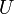$U$ is an open and convex subset of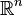$\mathbb R^n$ and if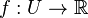$f : U \to \mathbb R$ is differentiable with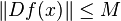$\|D f (x)\| \leq M$ for all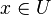$x \in U$ then we have that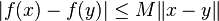$|f(x) - f(y)| \leq M \|x - y\|$ for all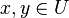$x, y \in U$.
We also proved the analogous statement if$f$ is Lipschitz continuous instead of having uniformly bounded derivative.
Lastly, we created a formulation for the problem if$U$ is star-shaped rather than convex.